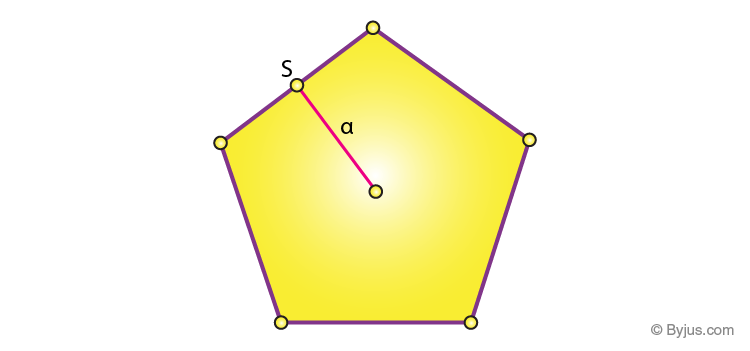# Area of a Pentagon Formula

The area of a pentagon formula is based on its sides and apothem length.

A pentagon is a five-sided polygon in geometry. It may be simple or self – intersecting in shape. The five angles present in the Pentagon are equal. A regular pentagon has all of the sides and angles are equal.

Pentagons can be regular or irregular and convex or concave. A regular pentagon is one with all equal sides and angles. Its interior angles measure 108 degrees and its exterior angles measure 72 degrees. An irregular pentagon is a shape that does not have equal sides and/or angles and therefore does not have specified angles. A convex pentagon is one whose vertices, or points, where the sides meet, are pointing outwards as opposed to a concave pentagon whose vertices point inwards. Imagine a collapsed roof of a house.

Now, the area of pentagon is derived by multiplying side and apothem length with (5/2). To learn more about the area of a pentagon along with the details of apothem and other related terms, check the linked article.## Area Formula for a Pentagon

The Area of a Pentagon Formula is,

 A = (5 ⁄ 2) × s × a

Where,

• “s” is the side of the Pentagon
• “a” is the apothem length

## Area of a Regular Pentagon Formula

If all the sides of a pentagon are equal in length, then it is a regular pentagon. The area of a regular pentagon is calculated by the formula:

$$\begin{array}{l}A=\frac{1}{4} \sqrt{5(5+2 \sqrt{5})} s^{2}\end{array}$$

where ‘s’ is the side length of a pentagon.

## Solved Examples on Area of Pentagon Formula

Question 1: Find the area of a pentagon of side 10 cm and apothem length 5 cm.

Solution: Given,

s = 10 cm

a = 5 cm

Area of a pentagon = A = (5 ⁄ 2) × s × a

= (5 ⁄ 2) × 10 × 5 cm2

= 125 cm2.

Question 2: Find the area of a regular pentagon, if the side length is 3cm.

Solution: Given, side length, s = 3 cm

$$\begin{array}{l}Area~of~a~regular~pentagon=\frac{1}{4} \sqrt{5(5+2 \sqrt{5})} s^{2}\end{array}$$

By putting the value of s = 3 cm, we get;

Area = 15.48 cm2

#### 1 Comment

1. MAHENDRA SINGH

Find the area of the given pentagon ABCDE in which each one of BF, CH and EG is perpendicular to AD such that AF = 9 cm, AG = 13 cm, AH = 19 cm, AD = 24 cm, BF = 6 cm, CH = 8 cm and EG = 9 cm